#### You may also like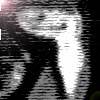### Clock Hands

This investigation explores using different shapes as the hands of the clock. What things occur as the the hands move.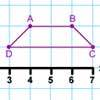### Transformation Tease

What are the coordinates of this shape after it has been transformed in the ways described? Compare these with the original coordinates. What do you notice about the numbers?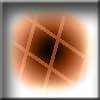### Penta Play

A shape and space game for 2, 3 or 4 players. Be the last person to be able to place a pentomino piece on the playing board.

# Coordinate Challenge

##### Age 7 to 11Challenge Level

We had lots of solutions to this problem but not many of you told us how you got your solutions. Luis and Freddie, who go to Newton Prep, sent in well-explained solutions. Here is what Luis wrote:

The first thing I worked out is that the letter at (4,2) is not symmetrical in any way, the only letter that isn't symmetrical in any way is the letter P.
Then I worked out that at (0,2) and (1,2) are the ends of the alphabet and had to be A and Z but I needed to know which one went where so I looked at the clues. One said that (0,2) and (2,0) have rotational symmetry which means that A is on (1,2) and Z is on (0,2).
Then I saw the clue that said that (3,3), (3,2) and (3,1) are all consecutive in the alphabet, because the A and the Z are already used, it has to be C, D and E because it says that (3,3) is made of only curved lines.
Then I saw that one of the clues said that (2,0) is only made of curved lines, so (2,0) is the S.
(4,2) is not symmetrical in any way so (4,2) is a P.
The first clue says that (1,1) and (1,3) have a vertical line of symmetry, another clue says that (1,1) also has a vertical line of symmetry, which means that (1,1) is a X and (1,3) is a Y, which leaves the B which is at (2,1) because a clue says that at (2,1) there is a letter with a horizontal line of symmetry.

Many children from Stradbroke Primary School sent an image of their solution. Here is George's: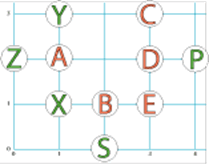Well done!

Rose and Thomas from Minterne Junior School wrote the following

We know that (1,1) = X because it has both horizontal and vertical symmetry.
A had to be (1,2) becuase it has vertical symmetry and is at one end of the alphabet.
Y was placed at (1,3) because it has vertical symmetry.
We knew that X, S and Z have rotational symmetry, but X has to be at (1,1), so Z was placed at (0,2) because it is also at the other end of the alphabet, and S at (2,0) becuase it has
curved lines.
We put C (3,3), D (3,2) and E at (3,1) because they are consecutive.
This works because C at (3,3) also had to consist of curved lines, and E (3,1) had
to have just straight lines.
Finally, we put B on (2,1) becuase it has horizontal symmetry, and P at (4,2) becuase it has no lines of symmetry.

We had the following solution come in from Writtle Junior School in Essex. Reggie, Esther and Emily want to share this:-

“I first worked out that [0,2] and [1,2] are A and Z but I needed to work what goes where , I saw [1,2] had vertical symmetry so [1,2]  is A so [0,2] is Z. P is not symmetrical in any way so [4,2] is P, S is the only one left with rotational symmetry and Z has taken [0,2] so S is [2,0]. [1,1] is symmetrical horizontally and vertically and X is both so [1,1] is X. Y is left with [1,3] because it is symmetrical vertically and none else are left symmetrical vertically so Y is [1,3]. E Is left with straight lines so E is [3,1]. E is at [3,1] and [3,1], [3,2] and [3,3] are consecutive in the alphabet so [3,2] is D and [3,3] is C. [2,1] is left and B is left [also horizontal like the clue] must be at [2,1].”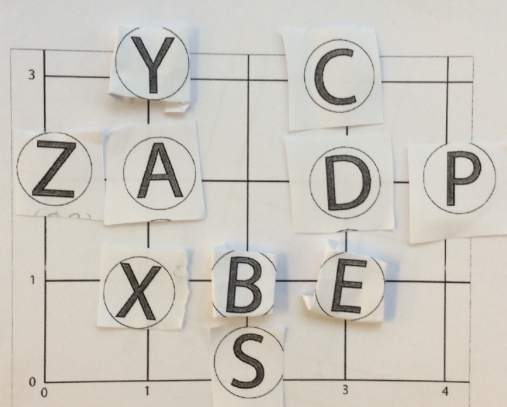Well done,all of you  for giving such clear descriptions as to how you came to your answer.